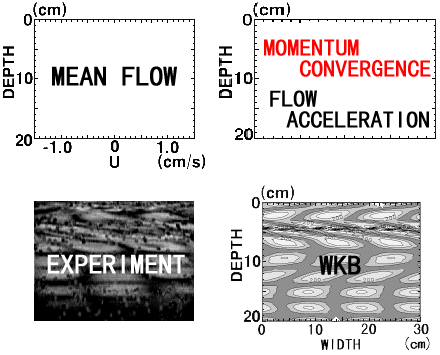Figure9 Flow in the QBO-like oscillation (upper-left).  Red line is the convergence of momentum flux, and Black line is the mean flow acceleration (upper-right). The internal gravity wave visualized by  the moíre method (lower-left). The result of WKB analysis (lower-right). The wave forcing frequency is 0.4s-1(period T = 15.7 s). Amplitude of piston is 13cm.